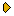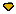HomeFor authorsSubmission statusCurrentArchive (English)ArchiveVolumes 81-92Volumes 61-80Volumes 21-40Volumes 1-20Volumes 41-60 Volume 60 Volume 59 Volume 58 Volume 57 Volume 56 Volume 55 Volume 54 Volume 53 Volume 52 Volume 51 Volume 50 Volume 49 Volume 48 Volume 47 Volume 46 Volume 45 Volume 44 Volume 43 Volume 42 Volume 41SearchVOLUME 59 (1994) | ISSUE 8 | PAGE 546
Scaling of correlation functions of velocity gradients in hydrodynamic turbulenceAs is demonstrated in [2, 3] in the limit of the infinitly large Reynolds number the correlation functions of the velocity predicted by Kolmogorov's 1941 theory (K41) are actually solutions of diagrammatic equations. Here we demonstrate that correlation functions of the velocity derivatives Vαυβ should possess scaling exponents which has no relation to K41 dimensional estimates, this phenomenon will be refered to as anomalous scaling. This result is proved on the diagrammatic language: we have extracted series of logarithmically diverging diagrams summation of which leads to renormalization of normal K41 dimensions. For a description of the scaling of various functions of Vavp an infinite set of primary fields 0„ with independent scaling exponents Δη can be introduced. Symmetry reasons enable us to predict relations between scaling of different correlation functions. Besides we formulate restrictions imposed on the structure of correlation functions due to the incompressibility condition. We also propose some tests enabling ones to check experimentally the conformal symmetry of the turbulent correlation functions. Further we demonstrate that the anomalous scaling behavior should reveal in the asymptotic behavior of correlations function of velocity differences and propose a way to obtain the anomalous exponents from the experiment.

Accesses: 55